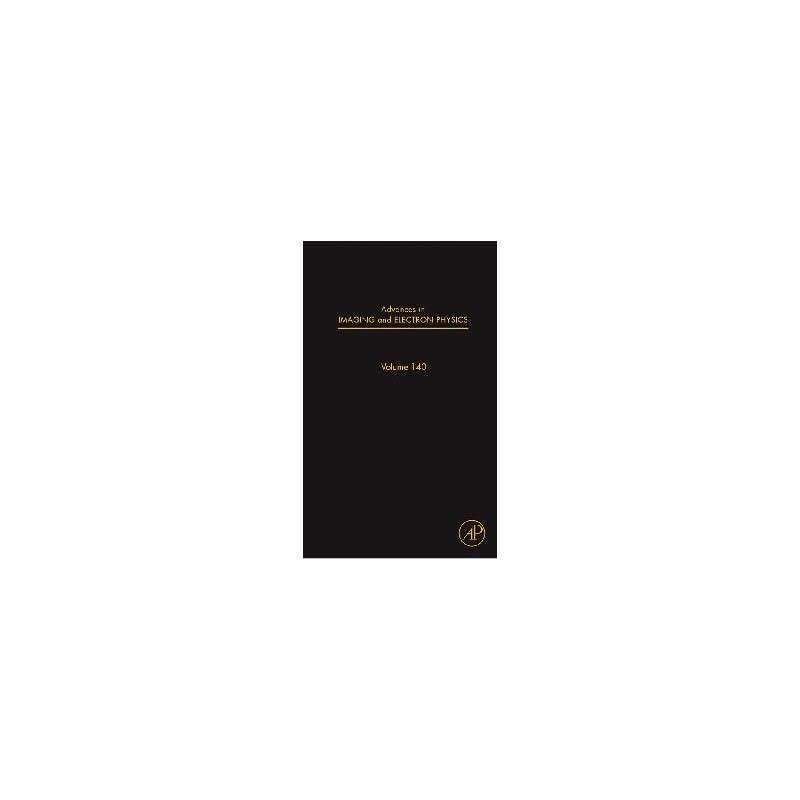﻿Zobacz większe

Mathematical Statistics with Applications

K.M. Ramachandran, Chris Tsokos

ID: 173642

Więcej informacji

Many students who do well in mathematics courses find it difficult to understand the concept of statistics. Mathematical Statistics and Its Applications is unique in that it presents the material with well-defined step by step procedures to solve real problems. This helps the students to approach problem solving in statistics in a logical manner.

This textbook provides a calculus based coverage of statistics and introduces students to methods of theoretrical statistics and their applications. It assumes no prior knowledge of statistics or probability theory but does require calculus. Most books at this level are written with eleaborate coverage of probability. This creates a problem for non statistics majors from various diciplines, who want to obtain a sound background in mathematical statstics and applications. The authors introduce the basic concepts of statistics with sound theoretical explanations. As statistics is basically an interdisciplinary applied subject, many applied examples and relevant exercises from different areas.

The book introduces many modern statistical computational and simulation concepts that are not covered in other texts; such as the Jackknife, bootstrap methods, the EM algorithms, and Markov chain Monte Carlo methods such as the Metropolis algorithm, Metropolis-Hastings algorthm and the Gibbs sampler.

▪ Step-by-step procedure to solve real problems, making the topic more accessible
▪ Exercises blend theory and modern applications
▪ Practical, real-world chapter projects
▪ Provides an optional section in each chapter on using Minitab, SPSS and SAS commands
▪ Student solutions manual, instructors manual and data disk available

Preface, Descriptive Statistics, Basic Concepts from Probability Theory, Additional Topics in Probability, Sampling Distributions, Point Estimation, Interval Estimation, Hypothesis Testing, Linear Regression Models, Design of Experiments, Analysis of variance, Bayesian Estimation and Inference, Nonparametric tests, Empirical Methods, Some issues in statistical applications- an overview, Appendices